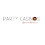## Sunday, July 4, 2021

### Java Collection #19 | Using Vector

Video demonstration

How to use java.util.Vector
How it is different from java.util.ArrayList
Different ways to add or remove elements.

In Java, a vector is a dynamic array that can grow or shrink in size based on the number of elements it contains. The Vector class in Java is a part of the java.util package and provides methods for manipulating dynamic arrays.

To use the Vector class in Java, we need to import the java.util package at the beginning. Here is an example:

import java.util.Vector;

public class MyClass {
public static void main(String[] args) {
// create a vector
Vector<String> vector = new Vector<String>();

// add elements to the vector

// get the size of the vector
int size = vector.size();
System.out.println("Size of vector: " + size);

// access an element in the vector
String element = vector.get(1);
System.out.println("Element at index 1: " + element);

// remove an element from the vector
vector.remove(2);

// print all elements in the vector
for (String s : vector) {
System.out.println(s);
}
}
}

In this example, we create a vector of strings, add elements to it, get the size of the vector, access an element in the vector, remove an element from the vector, and print all elements in the vector using a for-each loop. Note that the Vector class is synchronized, which means that it is thread-safe and can be used in a multi-threaded environment.

1.2.